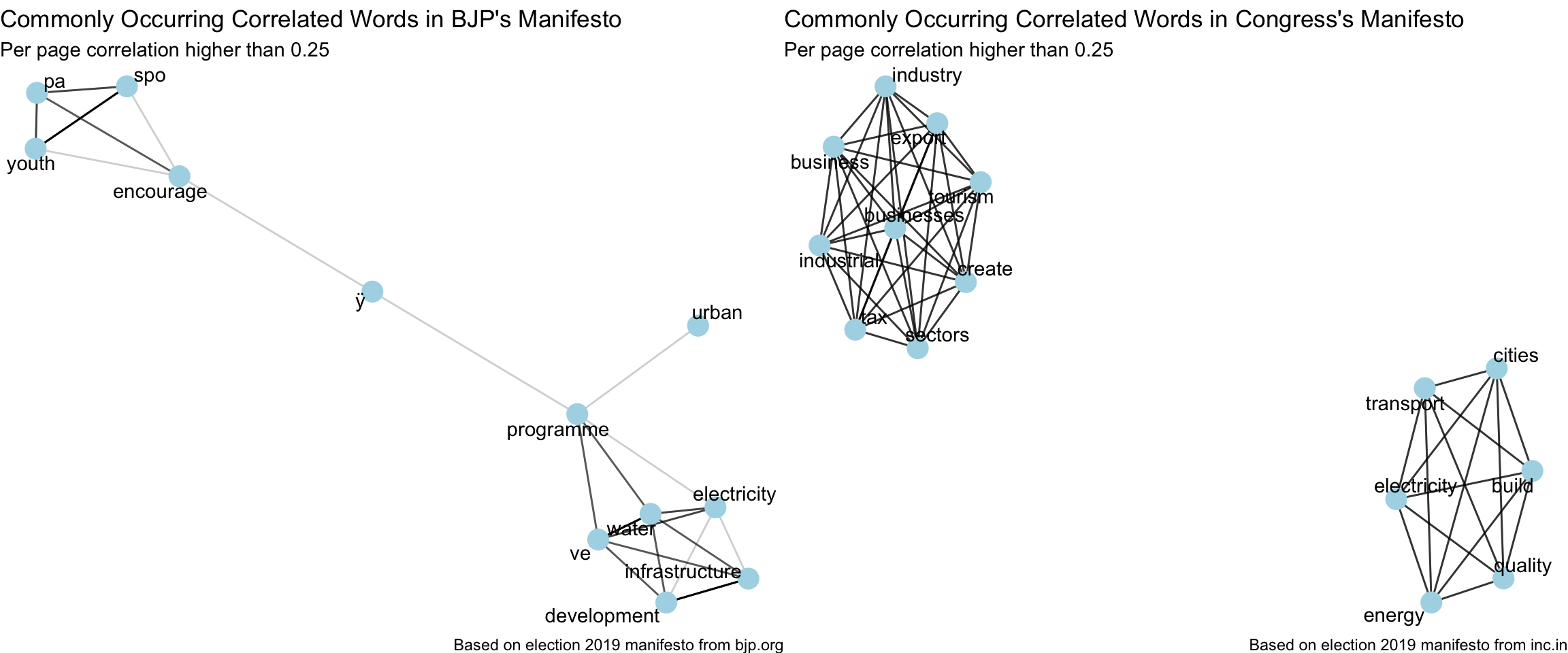# Introduction

With India’s 2019 General Elections around the corner, I thought it’d be a good idea to analyse the election manifestos of its 2 biggest political parties, BJP and Congress. Let’s use text mining to understand what each party promises and prioritizes.
In this part 3, I’ll explore the Employment and opportunities discussions in both manifestos.

# Analysis

``````rm(list = ls())
library(tidyverse)
library(pdftools)
library(tidylog)
library(hunspell)
library(tidytext)
library(ggplot2)
library(gridExtra)
library(scales)
library(reticulate)
library(widyr)
library(igraph)
library(ggraph)

theme_set(theme_light())
use_condaenv("stanford-nlp")``````

``````bjp_content <- read_csv("../data/indian_election_2019/bjp_manifesto_clean.csv")

## Employment and Opportunities

This topic is covered congress’ manifesto from Pages 6 to 8 of the pdf and in that of bjp’s from pages 20 to 22 and 27 to 28.

``````bjp_content %>%
filter(between(page, 20, 22) | between(page, 27, 28)) -> bjp_content

congress_content %>%
filter(between(page, 6, 8)) -> congress_content``````

## Common correlated words

``````plot_common_correlated_words <- function(df,
counts_quantile = 0.7,
correlation_threshold = 0.25,
stop_words_list = stop_words) {
set.seed(123)
df %>%
unnest_tokens(word, text) %>%
anti_join(stop_words_list) %>%
filter(n > stats::quantile(n, counts_quantile)) %>%
pairwise_cor(word, page, sort = TRUE) %>%
filter(correlation > correlation_threshold,
!str_detect(item1, "\\d"),
!str_detect(item2, "\\d")) %>%
graph_from_data_frame() %>%
ggraph(layout = "fr") +
geom_edge_link(aes(edge_alpha = correlation), show.legend = FALSE) +
geom_node_point(color = "lightblue", size = 5) +
geom_node_text(aes(label = name), repel = TRUE) +
theme_void() -> p

return(p)
}``````
``````bjp_content %>%
plot_common_correlated_words(stop_words_list = custom_stop_words,
counts_quantile = 0.85) +
labs(x = "",
y = "",
title = "Commonly Occurring Correlated Words in BJP's Manifesto",
subtitle = "Per page correlation higher than 0.25",
caption = "Based on election 2019 manifesto from bjp.org") -> p_bjp

congress_content %>%
plot_common_correlated_words(stop_words_list = custom_stop_words,
counts_quantile = 0.7) +
labs(x = "",
y = "",
title = "Commonly Occurring Correlated Words in Congress's Manifesto",
subtitle = "Per page correlation higher than 0.25",
caption = "Based on election 2019 manifesto from inc.in") -> p_congress

grid.arrange(p_bjp, p_congress, ncol = 2, widths = c(12,12))``````## Basic Search Engine

Lets build a cosine-similarity based simple search engine (instead of the basic keyword-based search that comes with the pdf document), in order to make these documents more easily searchable and gain context using most related lines in the manifestos for a given query. Using python’s scikit-learn for this.

``````from sklearn.feature_extraction.text import TfidfVectorizer, ENGLISH_STOP_WORDS
from sklearn.metrics.pairwise import linear_kernel

stopwords = ENGLISH_STOP_WORDS

vectorizer_bjp = TfidfVectorizer(analyzer='word', stop_words=stopwords, max_df=0.3, min_df=2)
vector_train_bjp = vectorizer_bjp.fit_transform(r["bjp_content\$text"])

vectorizer_congress = TfidfVectorizer(analyzer='word', stop_words=stopwords, max_df=0.3, min_df=2)
vector_train_congress = vectorizer_congress.fit_transform(r["congress_content\$text"])

def get_related_lines(query, party="bjp"):
if (party == "bjp"):
vectorizer = vectorizer_bjp
vector_train = vector_train_bjp
else:
vectorizer = vectorizer_congress
vector_train = vector_train_congress
vector_query = vectorizer.transform([query])
cosine_sim = linear_kernel(vector_query, vector_train).flatten()
return cosine_sim.argsort()[:-10:-1]``````
``get_related_lines <- py_to_r(py\$get_related_lines)``